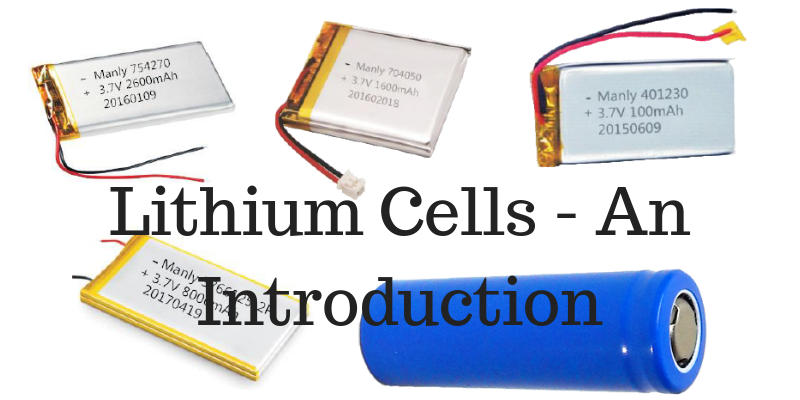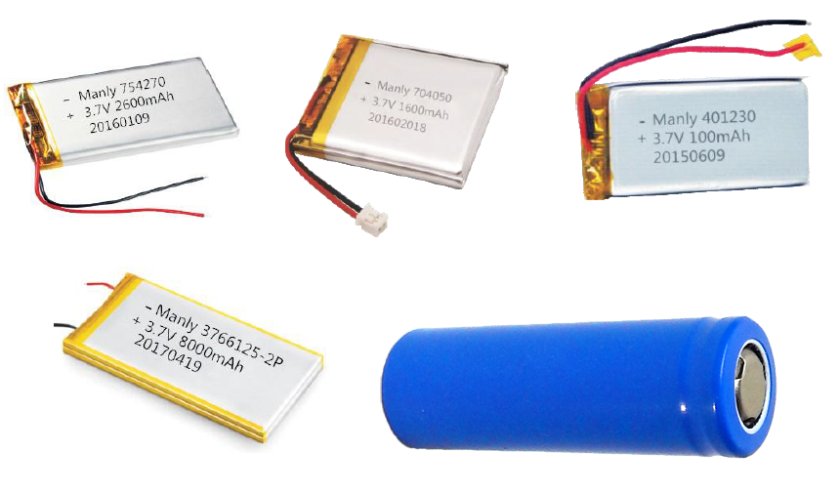Energy Storage Product Introduction Series

# An inroduction to Lithium Cells

Lithium Batteries are made of several Lithium cells. The cells are connected in combination of series and parallel to get the desired capacity and output. The cells come in different shapes such as cylindrical, prismatic and cubical. The cells are characterized by model, Nominal voltage, Nominal capacity (Ah), Standard Charge current (mA), internal resistance (mΩ). These specifications are described here.

Model

The model is usually the length and diameter of the cell in case of cylindrical cells. The cylindrical cells have models such as 18650, 16500, 14650, 14500, 14430, 14280, 26650, 26500, 22430. The first two numbers usually denote the diameter of the cell, while the last three denote the length of the cell in mm. For example, the cell 18650 has a diameter of 18mm and a length of 650mm.Nominal voltage

The nominal voltage is the voltage of the cell when charged. The nominal voltage of the lithium cell is 3.7V. These cells are unsually connected in series in order to get the desired voltage level of the battery.

# Types of Lithium Batteries

Nominal capacity (Ah)

The nominal capacity is the amount of energy that the battery can store. It is usllay mentioned in Ah. Higher the Ah, higher is the back up period. The cells are connected in parallel to get the desired capacity of the battery.

Standard Charge Current (mA)

The standard charge current is the value of the current that flows inside the cell.

Internal Resistance (mΩ)

The internal resistance is the resistance within the battery . This causes the voltage to drop when there is a flow of charge.

# The importance of Battery Management System

A typical specification will look like this

DescriptionValues
Model18650
Nominal Voltage (V)3.7
Nominal Capacity (mAh)3500
Diameter (mm ± 3)18
Height (mm ± 3)650
Standard Charge Current (mA)1750
Internal Resistance (mΩ)< 65

Call us